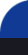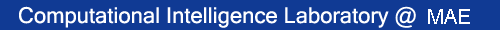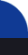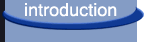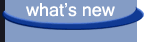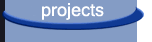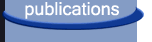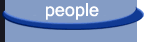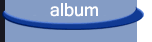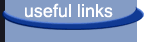Recent publications

 Y. Xia and J. Wang , “A recurrent neural network for nonlinear convex optimization subject to nonlinear inequality constraints ,” IEEE Transactions on Circuits and Systems - Part I : Regular Papers , vol. 51 , no. 7, pp. 1385-1394, 2004. Y. Xia, J. Wang , and L.-M. Fok, “ Grasping force optimization of multi-fingered robotic hands using a recurrent neural network ,” IEEE Transactions on Robotics and Automation , vol. 20, no. 3, pp. 549-554, 2004. L. Tian, J. Wang , and Z. Mao, “ Constrained motion control of flexible robot manipulators based on recurrent neural networks ,” IEEE Transactions on Systems, Man and Cybernetics - Part B: Cybernetics , vol. 34 , no. 3, pp. 1541-1552, 2004. D. Liu, S. Hu, and J. Wang , “ Global output convergence of a class of continuous-time recurrent neural networks with time-varying thresholds ,” IEEE Transactions on Circuits and Systems - Part II Express Briefs , vol. 51 , pp. 161-167, 2004. Y. Xia and J. Wang , “ A one-layer recurrent neural network for support vector machine learning ,” IEEE Transactions on Systems, Man and Cybernetics - Part B: Cybernetics , vol. 34 , no. 2, pp. 1261-1269, 2004. J. Cao and J. Wang , “ Absolute exponential stability of recurrent neural networks with time delays and Lipschitz-continuous activation functions ,” Neural Networks , vol. 17, no. 3, pp. 379-390, 2004. Y. Tan and J. Wang , “ A support vector machine with a hybrid kernel and minimal Vapnik-Chervonenkis dimension ,” IEEE Transactions on Knowledge and Data Engineering , vol. 16, no. 4, pp. 385-395, 2004. Y. Xia and J. Wang , “ A general projection neural network for solving optimization and related problems ,” IEEE Transactions on Neural Networks , vol. 15, pp. 318-328, 2004. Y. Zhang and J. Wang , “ Obstacle avoidance for kinematically redundant manipulators using a dual neural network ,” IEEE Transactions on Systems, Man and Cybernetics - Part B: Cybernetics , vol. 34, no. 1, pp. 752-759, 2004. X. Zhu, H. Ding, and J. Wang , “ Grasp analysis and synthesis based on a new quantitative measure ,” IEEE Transactions on Robotics and Automation , vol. 19, no. 6, pp. 942-953, 2003. S. Hu and J. Wang , “ Multilayer recurrent neural networks for on-line robust pole assignment ,” IEEE Transactions on Circuits and Systems - Part I: Fundamental Theory and Applications , vol. 50 , no. 11, pp. 1488-1496, 2003. Z. Zeng, J. Wang , and X. Liao, “ Global exponential stability of a general class of recurrent neural networks with time-varying delays ,” IEEE Transactions on Circuits and Systems - Part I: Fundamental Theory and Applications , vol. 50 , no. 10, pp. 1353-1358, 2003. J. Wang , “ Formation of machine cells and part families in cellular manufacturing systems using a linear assignment algorithm ,” Automatica , vol. 39, no. 9, pp. 1607-1615, 2003. Y. Zhu and J. Wang , “ Synthesis of force-closure grasps on 3-D objects based on the Q-distance ,” IEEE Transactions on Robotics and Automation , vol. 19, no. 4, pp. 669-679, 2003. X. Liao and J. Wang , “ Global dissipativity of continuous-time recurrent neural networks with time delay ,” Physical Review E , vol. 68, no. 1, pp. 016118.1-7, 2003. Y. Zhang, J. Wang, and Y. Xia, " A dual neural network for redundancy resolution of kinematically redundant manipulators subject to joint limits and joint velocity limits ," IEEE Transactions on Neural Networks , vol. 14, no. 3, pp. 658-667, 2003. X. Liao and J. Wang , " Algebraic criteria for global exponential stability of cellular neural networks with multiple time delays ," IEEE Transactions on Circuits and Systems - Part I: Fundamental Theory and Applications , vol. 50, no. 2, pp. 268-275, 2003. J. Cao and J. Wang, " Global asymptotic stability of a general class of recurrent neural networks with time-varying delays ," IEEE Transactions on Circuits and Systems - Part I: Fundamental Theory and Applications , vol. 50, no. 1, pp. 34-44, 2003. S. Hu and J. Wang , " Absolute exponential stability of a class of continuous-time recurrent neural networks ," IEEE Transactions on Neural Networks , vol. 14, no. 1, pp. 35-45, 2003. Y. Zhang, J. Wang , and Y. Xu, " A dual neural network for bi-criteria kinematic control redundant manipulators ," IEEE Transactions on Robotics and Automation , vol. 18, no. 6, pp. 923-931, 2002. Z. He, Y. Zhang, C. Wei, and J. Wang , " A multi-stage self-organizing algorithm combined transiently chaotic neural network for cellular channel assignment ," IEEE Transactions on Vehicular Technology , vol. 51, no. 6, pp. 1386-1396, 2002. S. Hu and J. Wang , " A gradient flow approach to on-line robust pole assignment for synthesizing output feedback control systems ," Automatica , vol. . 38, no. 11, pp. 1959-1968, 2002. Y. Zhang and J. Wang , " A dual neural network for constrained torque optimization of kinematically redundant manipulators ," IEEE Transactions on Systems, Man and Cybernetics - Part B: Cybernetics , vol. 32, no. 5, pp. 654-662, 2002. Y. Zhang, D. Jiang, and J. Wang , " A recurrent neural network for solving Sylvester equation with time-varying coefficients ," IEEE Transactions on Neural Networks , vol. 13, no. 5, pp. 1053-1063, 2002. S. Hu and J. Wang , " Global stability of a class of continuous-time recurrent neural networks ," IEEE Transactions on Circuits and Systems - Part I: Fundamental Theory and Applications , vol. 49, no. 9, pp. 1334-1347, 2002. S. Hu and J. Wang , " Global stability of a class of discrete-time recurrent neural networks ," IEEE Transactions on Circuits and Systems - Part I: Fundamental Theory and Applications , vol. 49, no. 8, pp. 1104-1117, 2002. M. Xiong, J. Wang , and P. Wang, " Differential-algebraic approach to linear programming ," Journal of Optimization Theory and Applications , vol. 114, no. 2, pp. 443-470, 2002. Y. Li and J. Wang , " Sequential blind extraction of instantaneously mixed sources ," IEEE Transactions on Signal Processing, vol. 50, no. 5, pp. 997-1006, 2002. S. Hu and J. Wang , " Global asymptotic stability and global exponential stability of continuous-time recurrent neural networks ," IEEE Transactions on Automatic Control , vol. 46, no. 5, pp. 802-807, 2002. Y. Zhang and J. Wang , " Global exponential stability of recurrent neural networks for synthesizing linear feedback control systems via pole assignment ," IEEE Transactions on Neural Networks , vol. 13, no. 3, pp. 633-644, 2002. Y. Xia, H. Leung, and J. Wang , " A projection neural network and its application to contained optimization problems ," IEEE Transactions on Circuits and Systems - Part I: Fundamental Theory and Applications , vol. 49, no. 4, pp. 447-458, 2002. S. Hu and J. Wang , " Global exponential stability of continuous-time interval neural networks ," Physical Review E , vol. 65, no. 3, pp.036133.1-036133.9, 2002. D. Jiang and J. Wang , " Augmented gradient flows for on-line robust pole assignment via state and output feedback ," Automatica , vol. 38, no. 2, pp. 279-286, 2002. Y. Tan and J. Wang , " Nonlinear blind source separation using higher-order statistics and a genetic algorithm ," IEEE Transactions on Evolutionary Computation , vol. 5, no. 6, pp. 600-612, 2001. X. Liang and J. Wang, " An additive diagonal stability condition for absolute exponential stability of a general class of neural networks ," IEEE Transactions on Circuits and Systems - Part I: Fundamental Theory and Applications , vol. 48, no. 11, pp. 1308-1317, 2001. Y. Zhang and J. Wang , " Recurrent neural networks for nonlinear output regulation ," Automatica , vol. 37, no. 8, pp. 1161-1173, 2001. Y. Xia and J. Wang , " Global asymptotic and exponential stability of a dynamic neural system with asymmetric connection weights ," IEEE Transactions on Automatic Control , vol. 46, no. 4, pp. 635-638, 2001. W. S. Tang and J. Wang , " A recurrent neural network for minimum infinity-norm kinematic control of redundant manipulators with an improved problem formulation and reduced architectural complexity ," IEEE Transactions on Systems, Man and Cybernetics - Part B: Cybernetics , vol. 31, no. 1, pp. 98-105, 2001. Y. Xia and J. Wang , " A dual neural network for kinematic control of redundant robot manipulators ," IEEE Transactions on Systems, Man and Cybernetics - Part B: Cybernetics , vol. 31, no. 1, pp. 147-154, 2001. Y. Tan, J. Wang , and J. M. Zurada, " Nonlinear blind source separation using a radial basis function network ," IEEE Transactions on Neural Networks , vol. 12, no. 1, pp. 124-134, 2001. S. Hu and J. Wang , " Quadratic stabilizability of a new class of linear systems with structural independent time-varying uncertainties ," Automatica , vol. 37, no. 1, pp. 51-59, 2001 S. Hu and J. Wang , " On stabilization of a new class of linear time-invariant interval systems via constant state feedback control ," IEEE Transactions on Automatic Control , vol. 45, no. 11, pp. 2106-2111, 2000. Y. Xia and J. Wang , " A discrete-time recurrent neural network for shortest-path routing ," IEEE Transactions on Automatic Control , vol. 45, no. 11, pp.2129-2135, 2000. X. Liang and J. Wang , " A recurrent neural network for nonlinear optimization with a continuously differentiable objective function and bound constraints ," IEEE Transactions on Neural Networks , vol. 11, no. 6, pp. 1251-1262, 2000. D. Jiang and J. Wang , " On-line learning of dynamic systems in the presence of model mismatch and disturbance ," IEEE Transactions on Neural Networks , vol. 11, no., 6, pp. 1272-1283, 2000. Y. Li, J. Wang , and J. M. Zurada, " Blind extraction of singularly mixed source signals ," IEEE Transactions on Neural Networks , vol. 11, no., 6, pp. 1413-1422, 2000. X. Li, S. K. Tso, and J. Wang , " Real-time tool condition monitoring using wavelet transform and fuzzy techniques ," IEEE Transactions on Systems, Man and Cybernetics - Part C: Applications and Reviews , vol. 31, no. 3, pp. 352-357, 2000. X. Liang and J. Wang , " Absolute exponential stability of neural networks with a general class of activation functions ," I IEEE Transactions on Circuits and Systems - Part I: Fundamental Theory and Applications , vol. 47, no. 8., pp. 1258-1263, 2000. Y. Xia and J. Wang , " Global exponential stability of recurrent neural networks for solving optimization and related problems ," IEEE Transactions on Neural Networks , vol. 11, no. 4, pp. 1017-1022, 2000. Y. Xia and J. Wang , " On the stability of globally projected dynamical systems, " Journal of Optimization Theory and Applications , vol. 106, no. 1, pp. 129-150, 2000. D. Jiang and J. Wang , " A recurrent neural network for on-line design of robust optimal filters ," IEEE Transactions on Circuits and Systems - Part I: Fundamental Theory and Applications , vol. 47, no. 6, pp. 921-926, 2000. Y. Xia and J. Wang ,, " A recurrent neural network for solving linear projection equations ," Neural Networks , vol. 13, no. 3, pp.337-350, 2000. X. Liang and J. Wang ,, " A proof of Kaszkurwicz and Bhara's conjecture on absolute stability of neural networks in two-neuron case ," IEEE Transactions on Circuits and Systems - Part I: Fundamental Theory and Applications , vol. 47, no. 4, pp. 609-611, 2000. W. S. Tang and J. Wang, " Two recurrent neural networks for joint torque optimization of kinematically redundant manipulators ," IEEE Transactions on Systems, Man and Cybernetics - Part B: Cybernetics , vol. 30, no. 1, pp. 120-128, 2000. J. Wang , Q. Hu, and D. Jiang, " A Lagrangian network for kinematic control of redundant robot manipulators ," IEEE Transactions on Neural Networks , vol. 10, no. 5, pp. 1123-1132, 1999. H. Ding and J. Wang , " Recurrent neural networks for minimum infinity-norm kinematic control of redundant manipulators ," IEEE Transactions on Systems, Man, and Cybernetics - Part A: Systems and Humans , vol. 29, no. 3, pp. 269-276, 1999. Y. Xia, J. Wang , and D. L. Hung, " Recurrent neural networks for solving linear inequalities and equations ," IEEE Transactions on Circuits and Systems - Part I: Fundamental Theory and Applications , vol. 46, no. 4, pp. 452-462, 1999. J. Wang , " A linear assignment clustering algorithm based on the least similar cluster representatives ," IEEE Transactions on Systems, Man, and Cybernetics - Part A: Systems and Humans , vol. 29, no. 1, pp. 100-104, 1999. D. Jiang and J. Wang , " A recurrent neural network for real-time semi-definite programming ," IEEE Transactions on Neural Networks , vol. 10, no. 1, pp. 81-93, 1999. Y. Xia and J. Wang , " A general methodology for designing globally convergent optimization neural networks ," IEEE Transactions on Neural Networks , vol. 9, no. 6, pp. 1331-1343, 1998. J. Wang , " Primal and dual neural networks for shortest-path routing ," IEEE Transactions on Systems, Man, and Cybernetics - Part A: Systems and Humans , vol. 28, no. 6, pp. 864-869, 1998. J. Wang and G. Wu, " A multilayer recurrent neural network for solving continuous-time algebraic Riccati equations ," Neural Networks , vol. 11, no. 5, pp. 939-950, 1998. J. Wang and Y. Xia, " Analysis and design of primal-dual assignment networks ," IEEE Transactions on Neural Networks , vol. 9, no. 1, pp. 183-194, 1998. J. Wang , " Recurrent neural networks for computing pseudoinverses of rank-deficient matrices ," SIAM Journal on Scientific Computing , vol.18, no. 5, pp. 1479-1493, 1997. J. Wang , " Primal and dual assignment networks ," IEEE Transactions on Neural Networks , vol. 8, no. 3, pp. 784-790, 1997. J. Wang and C. Roze, " Formation of machine cells and part families: a modified p-median formulation and a comparative study ," International Journal of Production Research , vol. 35, no. 5, pp. 1269-1286, 1997. J. Wang , " A recurrent neural network for solving the shortest path problem ," IEEE Transactions on Circuits and Systems - Part I: Fundamental Theory and Applications , vol. 43, no. 6, pp. 482-486, 1996. J. Wang and G. Wu, " A multilayer recurrent neural network for on-line synthesis of minimum-norm linear feedback control systems via pole assignment ," Automatica , vol. 32, no. 3, pp. 435-442, 1996. J. Wang , " Analysis and design of an analog sorting network ," IEEE Transactions on Neural Networks , vol. 5, no. 4, pp. 962-971, 1995. J. Wang , " A deterministic annealing neural network for convex programming ," Neural Networks , vol. 7, no. 4, pp. 629-641, 1994. J. Wang , "Artificial neural networks versus natural neural networks: a connectionist paradigm for preference assessment," Decision Support Systems , vol. 11, no. 5, pp. 415-429, 1994. J. Wang , “ Analog winner-take-all neural networks for determining maximum and minimum signals ,” International Journal of Electronics , vol. 77, no. 3, pp. 355-367, 1994. J. Wang and B. Malakooti, "Characterization of training errors in supervised learning using gradient-based learning rules," Neural Networks , vol. 6, no. 8, pp. 1073-1087, 1993. J. Wang , " Analysis and design of a recurrent neural network for linear programming ," IEEE Transactions on Circuits and Systems - Part I: Fundamental Theory and Applications , vol. 40, no. 9, pp. 613-618, 1993.